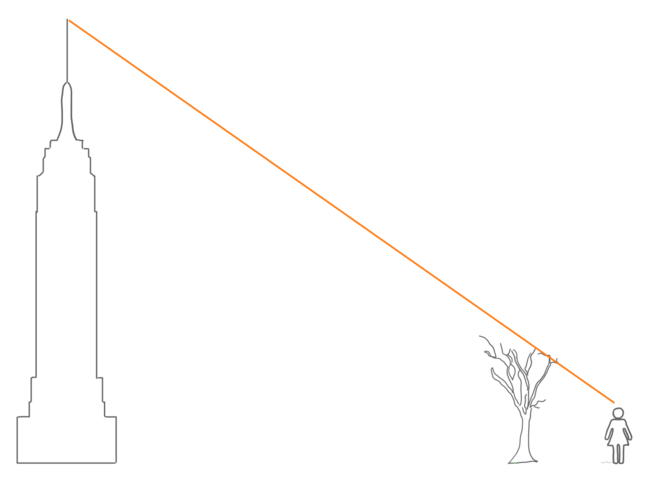## Want to keep learning?

This content is taken from the University of Padova's online course, Advanced Precalculus: Geometry, Trigonometry and Exponentials. Join the course to learn more.
2.2

Skip to 0 minutes and 12 seconds Hello. Welcome to the section of exercises of the Week 2. Remember to try to solve yourself the exercises before watching the videos and before looking at the solutions that you can find in the PDF file at the bottom of the page. OK. It’s your turn on trigonometry by triangles. Good. We have the following exercise. We have a triangle, ABC.

Skip to 0 minutes and 45 seconds In red, we have the sides and the angle that we know, you see. And we would like to compute the area of this triangle. As you have seen in the Francis’ video, the area of our triangle is equal to 1 over 2 times b times c times the sine of the angle formed by the sides b and c, that is the angle in A. OK. We know b. We know c. We have to compute the sine of A. Good. First of all, let us remember that by the law of sines, we have that the sine of B over b is equal to the sine of C over c.

Skip to 1 minute and 40 seconds And then we can immediately get that the sine of C is equal to c over b times the sine of B. OK. And now we know that c is 6. We know that b is equal 2 times the square root of 3.

Skip to 2 minutes and 9 seconds The angle B is 30 degrees. Therefore the sine of B is equal to 1 over 2. And then what we get, we get the square root of 3 over 2. Good. But now, of course, there are two possibilities for our angle C. Indeed, C prime equal to 60 degrees is one possibility. And we have another possibility, which is C second equal to 120 degrees. And both these angles have sine equal to the square root of 3/2. Indeed, both the possibilities happens. Precisely, we have these two situations. This is the situation where the angle in C is 60 degrees. This is the situation where the angle in C is 120 degrees. OK.

Skip to 3 minutes and 29 seconds Therefore, we have two triangles satisfying the data of our exercise. OK. In the first case now, knowing that the sum of the internal angles in a triangle is 180, then in the first case we immediately get that the angle A prime is equal to 180 degrees minus the angle in B, which is 30 degrees, minus the angle C prime, which is minus– which is 60 degrees. And then what we get, we get 90 degrees. In the second case, we have A second equal to 180 degrees, again minus the angle in B, which is 30 degrees, and now minus the angle C second, which is 120 degrees. OK. And we get 30 degrees. Good.

Skip to 4 minutes and 41 seconds We have then– in these two cases, we have to compute the two possible areas. OK. In the first case, the area is equal to what? We have 1 over 2 times b, which is 2 times the square root of 3, c, which is 6, times the sine of A prime. But the sine of 90 degrees is 1. And this is the area A prime. OK. In this second case, what do we have? The area, we call it A second, is equal again to 1 over 2 times 2 times the square root of 3 times 6 times now what? The sine of 30 degrees. And the sine of 30 degrees is equal to 1 over 2.

Skip to 5 minutes and 42 seconds Therefore, we get in the first case 6 times the square root of 3. And in the second case, we get 3 times the square root of 3. OK. Thank you very much for your attention.

# It's your turn on trigonometry by triangles

Do your best in trying to solve the following problems. In any case some of them are solved in the video and all of them are solved in the pdf file below.

### Exercise 1.

Assume a triangle $$ABC$$ with sides $$a$$, $$b$$, $$c$$ has $$b=2\sqrt 3$$, $$c=6$$ and the angle in $$B$$ equal to $$30^o$$. What could be its area?

### Exercise 2.

Lina is watching the Empire State Building. Between her and the skyscraper there is a tree whose height is approximately equal to 3 meters. She notices that the top of the tree and the top of the skyscraper are on the same line of sight (the line from the eye of Lina and the top of the tree or of the skyscraper):The distance between Lina and the tree is equal to 5 meters; the distance between the tree and the base of the skyscraper is equal to 1455 meters. Which is the height of the skyscraper if Lina is 1.70 meters high?